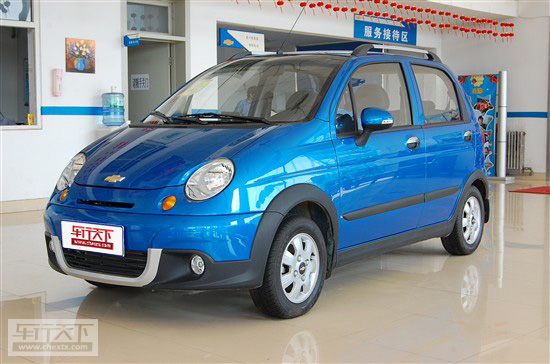## 沙尘暴肆虐 沙尘暴侵害车漆腐蚀后的防护

2014年07月30日 14:45 来源：www.chextx.com 超过：次关注

许多车主只重视发动机的保养，而忽视车漆表面的护理，冬季干燥的气候，强紫外线的福射及沙尘爆侵害，是车漆腐蚀的主要根源，加上北京风沙多，不适当的洗车、打蜡会使漆面变污，失去光泽。那么怎样能使漆面尽快复原呢?首先要根据车漆表面损伤程度的不同，选用不同的方法，损伤较重的最好做封釉处理，它的工艺中，含有抛光、修复、增艳等功能，能使车漆焕燃一新，封釉后的车就象有了一层隐形车衣，抗紫外线、酸减、风沙，甚至不怕火烧，但费用较贵。损伤中度的可做CTS膜处理。损伤轻度的可做镀膜处理。

沙尘爆会通过汽车的每一个缝隙进入您的车内，使车的仪表台、座椅等蒙上一层尘埃，这些表面的灰尘不会对车有较大的损害，只是影响清洁而已，但落在空调滤芯的尘埃可就麻烦了，空调启动时，就会顺着风道进入车内，使空调系统污染，风道变脏。因此，风沙过后应立刻更换空调滤芯或使用压缩空气对空调滤芯进行清理，冬季过后没有对空调系统养护的车辆，还要对整个空调系统进行全面清洗，杀灭细菌，彻底清除污染源，并检查调试空调系统，迎接夏天的来临。

沙尘暴易发区选抗沙尘性车蜡

车主应该根据车辆的具体情况来选择车蜡。

首先，沿海地区应用防盐雾功能较强的车蜡;化学工业区应选用防酸雨功能较强的车蜡;多雨地区应选用防水性能优良的车蜡;光照好的地区应选用防紫外线、抗高温性能好的车蜡;沙尘暴易发地区应选用抗沙尘性能好的车蜡。

其次，如果车辆经常在泥泞、沙石、多尘等恶劣路面环境下行驶，就应选用保护功能较强的硅酮树脂蜡。

其三，如夏季一般光照较强，应选用防高温、防紫外线能力强的车蜡。

其四，新车或较新漆面可选用新车专用蜡等上光蜡;已有损伤的漆面可选用软蜡，同时进行抛光和打蜡。

最后，在使用色彩蜡时，要选择与车漆颜色一致的车蜡。另外，在进行研磨处理时，白色及浅色车可使用研磨作用较强的研磨剂，而深色和金属色车漆多为透明漆，应尽量选用较柔和的研磨剂。

﻿
• 快速找车
• 选择品牌
• 选择品牌
• A  奥迪
• A  阿斯顿·马丁
• A  阿尔法·罗密欧
• B  宝沃
• B  布加迪
• B  巴博斯
• B  保时捷
• B  宾利
• B  奔驰
• B  宝马
• B  本田
• B  别克
• B  标致
• B  比亚迪
• B  宝骏
• B  北汽制造
• B  北汽新能源
• B  北汽幻速
• B  北汽威旺
• B  北京汽车
• B  奔腾
• B  北汽绅宝
• B  北汽昌河
• C  长安欧尚
• C  长安
• C  长安凯程
• C  长城
• D  大众
• D  道奇
• D  DS
• D  东南
• D  东风风神
• D  东风风行
• D  东风小康
• D  东风风度
• D  东风
• F  福特
• F  丰田
• F  菲亚特
• F  法拉利
• F  福田
• F  福迪
• F  福汽启腾
• G  观致
• G  广汽传祺
• G  广汽吉奥
• G  GMC
• H  红旗
• H  汉腾汽车
• H  哈弗
• H  哈飞
• H  海格
• H  海马
• H  华颂
• H  黄海
• H  华泰
• H  恒天
• J  几何汽车
• J  捷达
• J  吉利汽车
• J  捷豹
• J  Jeep
• J  江淮
• J  江铃
• J  金杯
• J  九龙
• J  金旅
• K  凯翼
• K  凯迪拉克
• K  克莱斯勒
• K  科尼塞克
• K  卡威
• K  开瑞
• L  路虎
• L  林肯
• L  劳斯莱斯
• L  兰博基尼
• L  雷克萨斯
• L  铃木
• L  领克
• L  雷诺
• L  理念
• L  力帆
• L  莲花汽车
• L  猎豹
• L  路特斯
• L  陆风
• M  马自达
• M  MG
• M  MINI
• M  玛莎拉蒂
• M  摩根
• M  迈凯轮
• N  纳智捷
• O  欧拉
• O  欧宝
• O  讴歌
• O  欧朗
• Q  奇瑞
• Q  起亚
• Q  启辰
• R  日产
• R  荣威
• R  瑞麒
• S  SERES赛力斯
• S  三菱
• S  斯威汽车
• S  萨博
• S  smart
• S  斯柯达
• S  斯巴鲁
• S  思铭
• S  双龙
• S  上汽大通
• S  双环
• T  特斯拉
• T  腾势
• W  蔚来
• W  沃尔沃
• W  WEY
• W  五菱汽车
• W  五十铃
• W  威兹曼
• W  威麟
• X  现代
• X  雪佛兰
• X  星途
• X  雪铁龙
• X  小鹏汽车
• X  西雅特
• Y  一汽
• Y  英菲尼迪
• Y  英致
• Y  依维柯
• Y  野马汽车
• Y  永源
• Z  众泰
• Z  中华
• Z  中兴
• Z  知豆
• 选择车系
• 选择车系
• 车型对比
• 选择品牌
• 选择品牌
• A  奥迪
• A  阿斯顿·马丁
• A  阿尔法·罗密欧
• B  宝沃
• B  布加迪
• B  巴博斯
• B  保时捷
• B  宾利
• B  奔驰
• B  宝马
• B  本田
• B  别克
• B  标致
• B  比亚迪
• B  宝骏
• B  北汽制造
• B  北汽新能源
• B  北汽幻速
• B  北汽威旺
• B  北京汽车
• B  奔腾
• B  北汽绅宝
• B  北汽昌河
• C  长安欧尚
• C  长安
• C  长安凯程
• C  长城
• D  大众
• D  道奇
• D  DS
• D  东南
• D  东风风神
• D  东风风行
• D  东风小康
• D  东风风度
• D  东风
• F  福特
• F  丰田
• F  菲亚特
• F  法拉利
• F  福田
• F  福迪
• F  福汽启腾
• G  观致
• G  广汽传祺
• G  广汽吉奥
• G  GMC
• H  红旗
• H  汉腾汽车
• H  哈弗
• H  哈飞
• H  海格
• H  海马
• H  华颂
• H  黄海
• H  华泰
• H  恒天
• J  几何汽车
• J  捷达
• J  吉利汽车
• J  捷豹
• J  Jeep
• J  江淮
• J  江铃
• J  金杯
• J  九龙
• J  金旅
• K  凯翼
• K  凯迪拉克
• K  克莱斯勒
• K  科尼塞克
• K  卡威
• K  开瑞
• L  路虎
• L  林肯
• L  劳斯莱斯
• L  兰博基尼
• L  雷克萨斯
• L  铃木
• L  领克
• L  雷诺
• L  理念
• L  力帆
• L  莲花汽车
• L  猎豹
• L  路特斯
• L  陆风
• M  马自达
• M  MG
• M  MINI
• M  玛莎拉蒂
• M  摩根
• M  迈凯轮
• N  纳智捷
• O  欧拉
• O  欧宝
• O  讴歌
• O  欧朗
• Q  奇瑞
• Q  起亚
• Q  启辰
• R  日产
• R  荣威
• R  瑞麒
• S  SERES赛力斯
• S  三菱
• S  斯威汽车
• S  萨博
• S  smart
• S  斯柯达
• S  斯巴鲁
• S  思铭
• S  双龙
• S  上汽大通
• S  双环
• T  特斯拉
• T  腾势
• W  蔚来
• W  沃尔沃
• W  WEY
• W  五菱汽车
• W  五十铃
• W  威兹曼
• W  威麟
• X  现代
• X  雪佛兰
• X  星途
• X  雪铁龙
• X  小鹏汽车
• X  西雅特
• Y  一汽
• Y  英菲尼迪
• Y  英致
• Y  依维柯
• Y  野马汽车
• Y  永源
• Z  众泰
• Z  中华
• Z  中兴
• Z  知豆
• 选择车系
• 选择车系
• 选择车型
• 选择车型
• 意见反馈